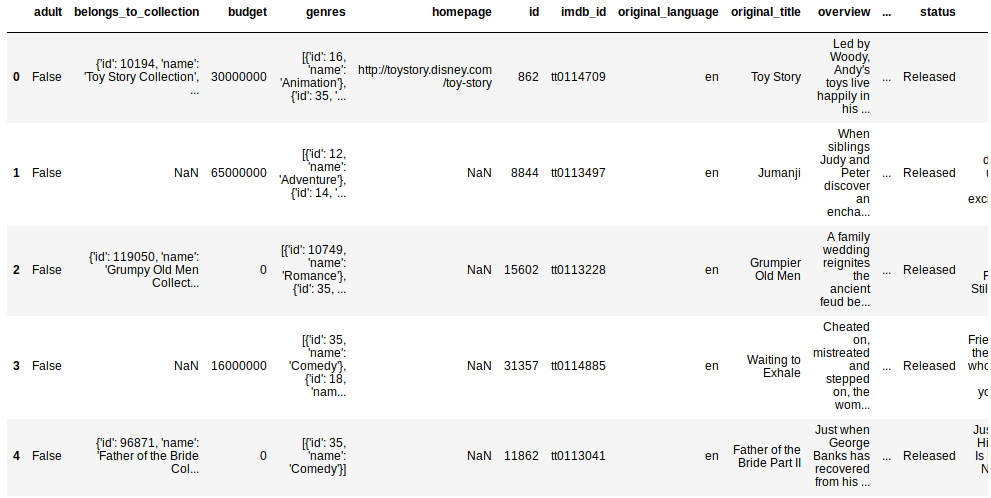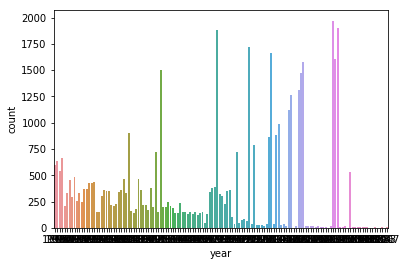## Recommendation System using Natural Language Processing Technique in Python

##### In this part of Learning Python we Cover Natural Language Processing In Python
Written by |11-May-2019 | 0 Comments | 321 Views

In this section, we will see how to make a recommendation system of movies by using natural language processing. There are multiple types of recommendation system normally that market is using currently.

• Content-Based Filtering: In this filtering, we use the name of the movies and description of the movies and then find the similarity by using the textual data. In this technique, the rules of natural language processing will be used. Today we will be using this technique.
• Collaborative Technique: It is a technique in which we recommend the movie to the user by likes, dislikes or rating of other users.
• Hybrid Filtering: Hybrid filtering is the technique in which both content-based and collaborative are merged to form one filtering technique.

So today we will be doing the content-based technique and for which we will be using the dataset from kaggle. Now lets deep dive into the code. We will first import the essential modules.

`import pandas as pd`
`import numpy as np`
`from ast import literal_eval`
`from sklearn.feature_extraction.text import TfidfVectorizer, CountVectorizer`
`from sklearn.metrics.pairwise import linear_kernel, cosine_similarity`
`import warnings`
`warnings.simplefilter('ignore')`

Pandas for importing the CSV and for data manipulation pandas will be used. Eval is the library that converts the string to the python object. For example, if we have a string that has a list like characters than eval will convert to the real list. It evaluates the string to the real python object

The other module that we are going to import is vectorizer. A computer does not understand what text is, so we have to convert the text to vectors and for that this is the best method.

Then we will use the similarity method to find the similarity between the text.

Now we will import the CSV files so that we can do the further implementation.

`movie = pd.read_csv('movie.csv')`
`links = pd.read_csv('links_small.csv')`

Now after that, the data looks like this:Figure 1

There are many features in the movie data set so. First, we have to make the clean. But what we have to clear. We can see that genre is given in the list form. But the type of the genre is a string. To parse the data and iterate over the list and dictionary we first have to evaluate the data into the python object. So the following action should be made.

`movie['genres'] = movie['genres'].fillna('[]').apply(literal_eval).apply(lambda x:[i['name'] for i in x] if isinstance(x,list) else [])`

`movie['year'] = pd.to_datetime(movie['release_date'], errors='coerce').apply(lambda x:str(x).split('-') if x!=np.nan else np.nan)`

In the first line, we have to change the object type of genre from string to list object. We first fill the null value to an empty string. After that, we converted the string date to the actual date time format of python. By the date time, we can know how much movies are released in which year.

`sns.countplot(movie['year'])`Figure 2

This the plot based on movies released in different years. Now we will link the two CSV data by the id. In this way, we can make the size of the data of the movie smaller because the data is too much broad and it will consume much memory.

`links = links[links['tmdbId'].notnull()]['tmdbId'].astype('int')`
`movie['id'] = movie['id'].astype('int')`

In the above line of code, we have converted the id data to an integer so we can easily natch the ids from the 2 data source.

`small= movie[movie['id'].isin(links)]`
`small['tagline'] = small['tagline'].fillna('')`
`small['description'] = small['overview']+movie['tagline']`
`small['description'] = small['description'].fillna('')`

Now we will make a new data source that only includes the data which is both presents in the links and movies data. Moreover, then we make a description that includes the tagline of the movie and the overview of the movie. Which makes the total description of the data. The data looks like as following:

 1        When siblings Judy and Peter discover an encha... 2        A family wedding reignites the ancient feud be... 3        Cheated on, mistreated and stepped on, the wom... 4        Just when George Banks has recovered from his ... 5        Obsessive master thief, Neil McCauley leads a ... 6        An ugly duckling having undergone a remarkable... 7        A mischievous young boy, Tom Sawyer, witnesses... 8        International action superstar Jean Claude Van... 9        James Bond must unmask the mysterious head of ... 10       Widowed U.S. president Andrew Shepherd, one of...

It contains all the data that we can use for the extraction of data. Now to make these descriptions understandable to the computer we have to vectorize the data as follows:

`tf = TfidfVectorizer(analyzer='word',ngram_range=(1, 4),min_df=0, stop_words='english')`
`matrix = tf.fit_transform(small['description'])`

If we try to print the matrix following output can be seen.

`print(matrix)`

 Output: <9098x635391 sparse matrix of type ''         with 849580 stored elements in Compressed Sparse Row format>

We can see that the data is in compressed form. Now we will calculate the similarity by using cosine similarity.

`sim = cosine_similarity(matrix, matrix)`

The output of this code is as follows:

 array([[0.        , 0.        , 0.        , ..., 0.        , 0.        ,         0.        ],        [0.        , 1.        , 0.00715858, ..., 0.00185797, 0.        ,         0.        ],        [0.        , 0.00715858, 1.        , ..., 0.        , 0.        ,         0.        ],        ...,        [0.        , 0.00185797, 0.        , ..., 1.        , 0.        ,         0.        ],        [0.        , 0.        , 0.        , ..., 0.        , 0.        ,         0.        ],        [0.        , 0.        , 0.        , ..., 0.        , 0.        ,         0.        ]])

We can see that the data is too much sparsed.

`small = small.reset_index()`
`titles = small['title']`
`ind = pd.Series(small.index, index=titles)`

`def Get_Recommendation(title):`
`    idd = ind[title]`
`    scores = list(enumerate(sim[idd]))`
`    scores = sorted(scores, key=lambda x: x, reverse=True)`
`    scores = scores[1:20]`
`    movie_indices = [i for i in sim_scores]`
`    `
`    return titles.iloc[movie_indices]`

We then compute the similarity, we sort the 20 maximum or closely reside data. Now we will call the function, and we will see the results:

`Get_Recommendation('Jumanji')`

The output is as follows:

 8888                        Pixels 8607       Guardians of the Galaxy 8153                Wreck-It Ralph 6391                    Stay Alive 3195            Dungeons & Dragons 8669                         Ouija 5802               Comfort and Joy 5355      Night of the Living Dead 4081     The Giant Spider Invasion 6322                 Grandma's Boy 8210              Would You Rather 1644                     Peter Pan 2080                      eXistenZ 6544             Sleeping Dogs Lie 6284    Zathura: A Space Adventure 4172                    Rollerball 8409                  Ender's Game 1618                   BASEketball 7307                         Gamer

These are all movies that are close to Jumanji.